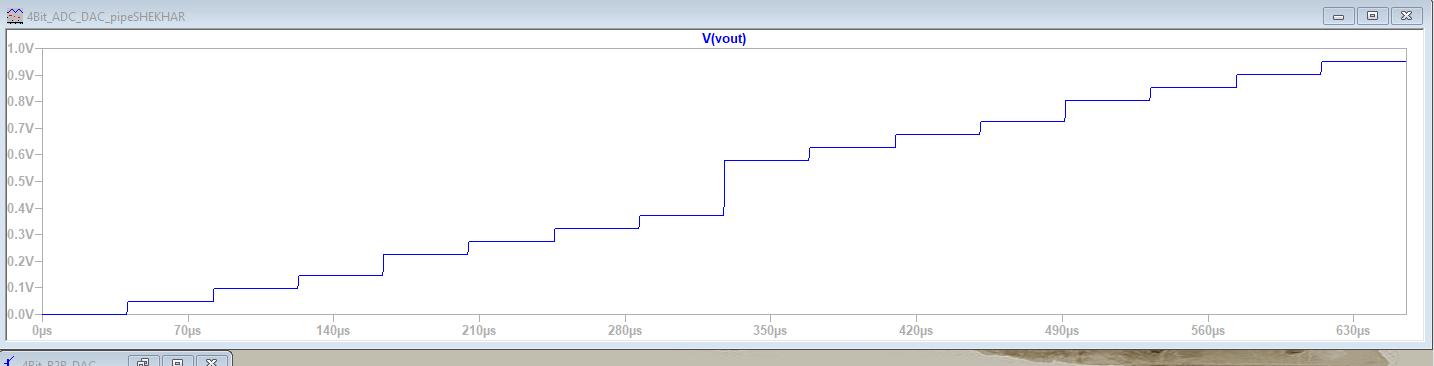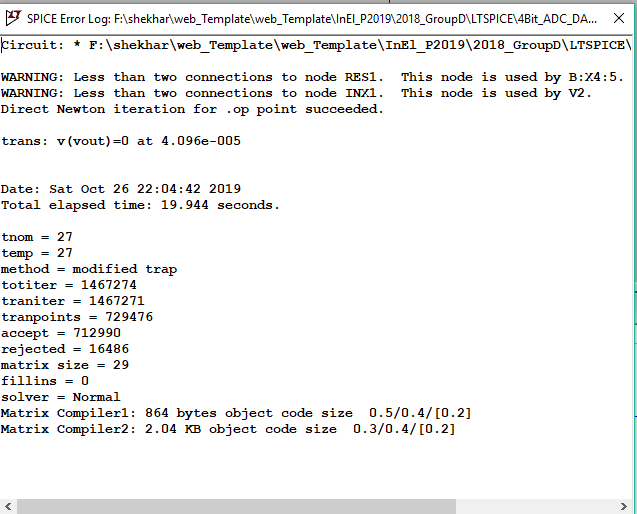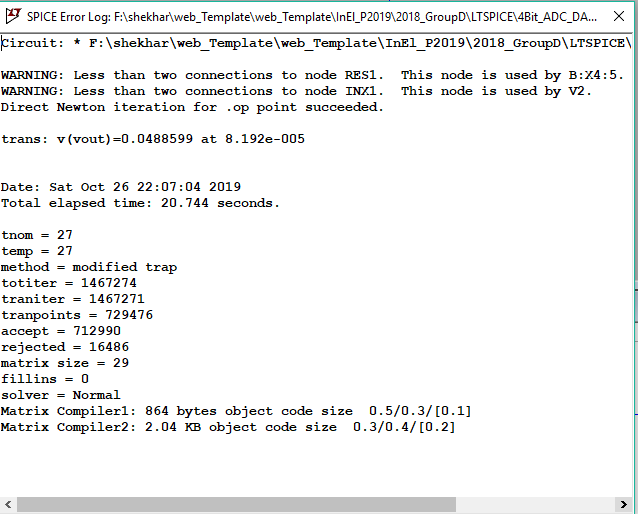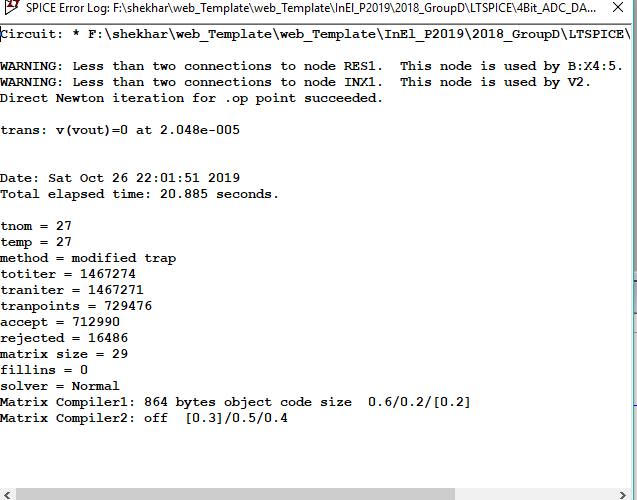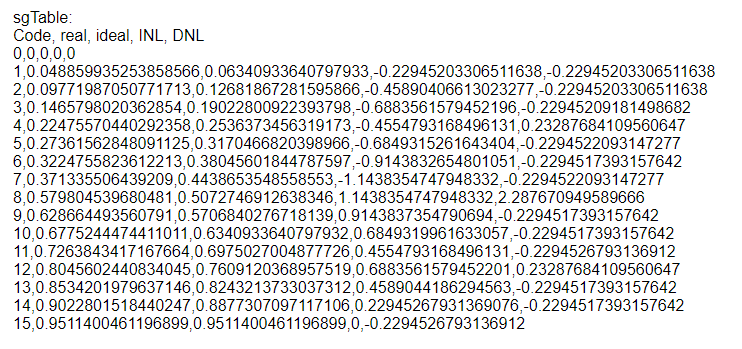# OVERVIEW

• MOTIVATION
• STARTING HTML
• SIMULATION OF RC LOW PASS FILTER IN LTSPICE
• SIMULATION OF IDEAL 4 BIT IDEAL ADC DAC
• INL DNL FFT ANALYIS OF ADC DAC
• SIMULATION OF ADC DAC WITH 4 BIT R2R CIRCUIT
• ANALYSIS OF LTSPICE SIMULATION FILES WITH .MEASURE STATEMENTS
• CONCLUSION

# Motivation

Options:
• Word, Powerpoint
• PDF
• HTML, JavaScript
Benfits:
• Everywhere, all devices fast access
• No conversion of documents
• Interactive elements

# STARTING HTML

• Unzip folder
• OPEN WEB FILE WITH NOTE++
• Edit required information

# Simulation of rc low pass filter in ltspice

 OPEN LT SPICE Design a rc low pass filter Provide value for R ,c and Voltage source Simuate with .op,.tran,.DC,and .Ac Corner frequency was found 32hz.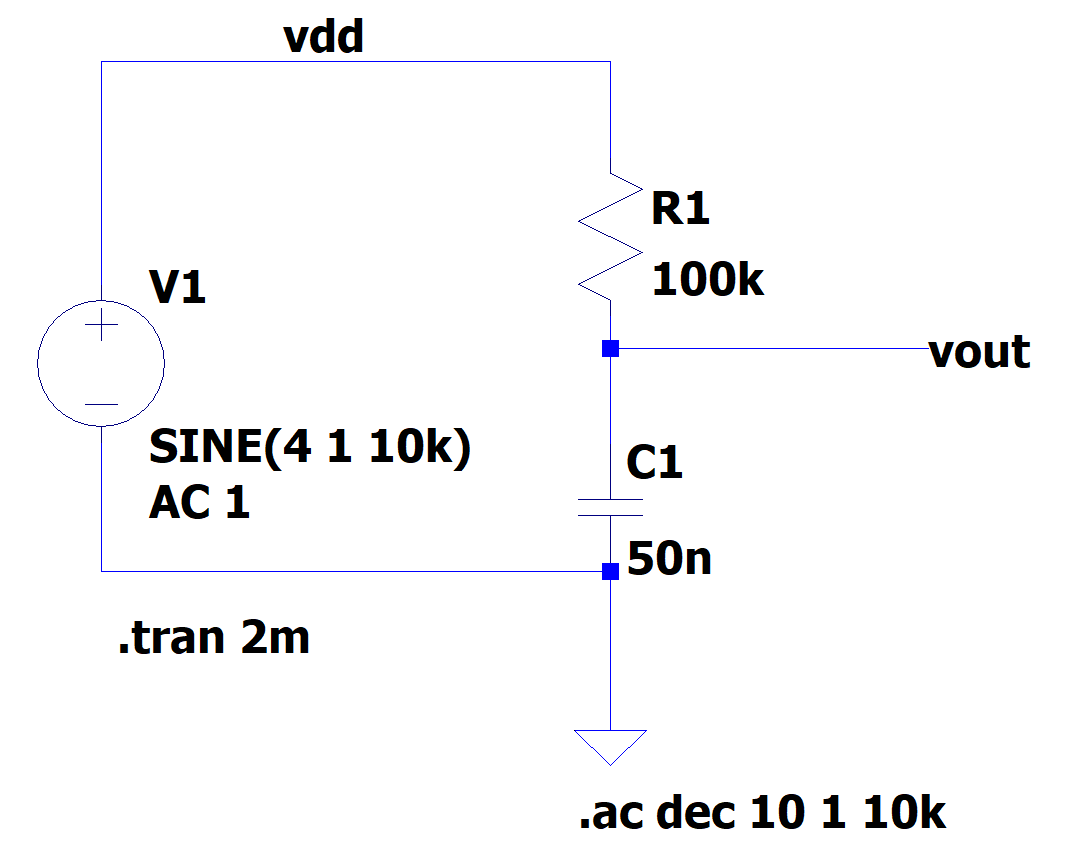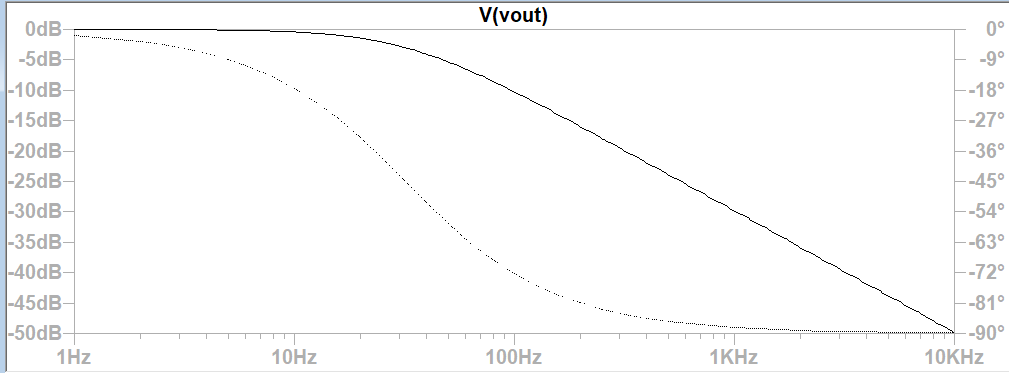# Simulation of 4-bit ideal ADC DAC

 Download the ADC and DAC circuit Provide the source Disable ramp by using X Disable one source at once by using X Doing FFT in 3rd fig. we found that output signal is very noisy In 4th fig. we see that all the values are evenly distributed and step size is fixed.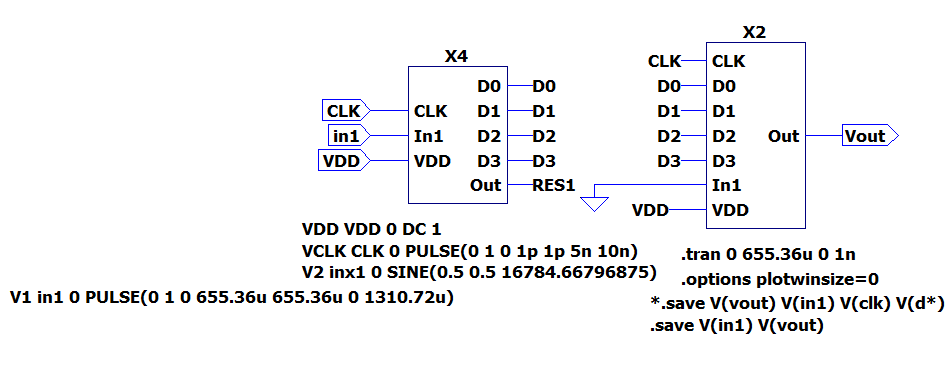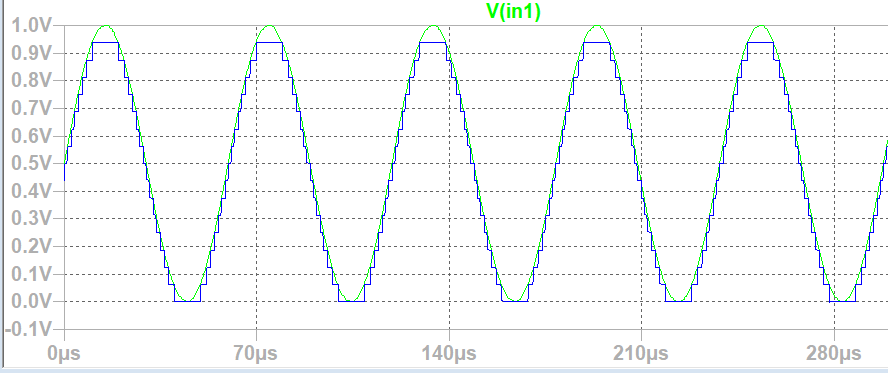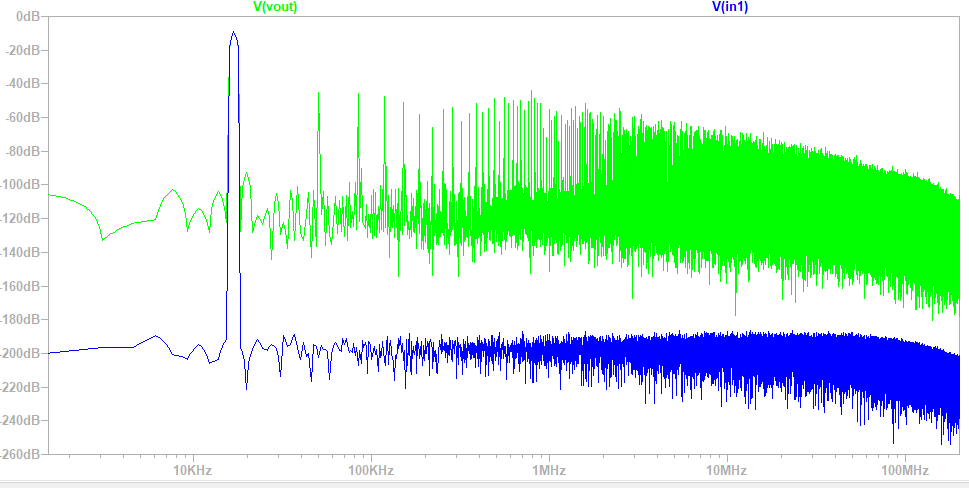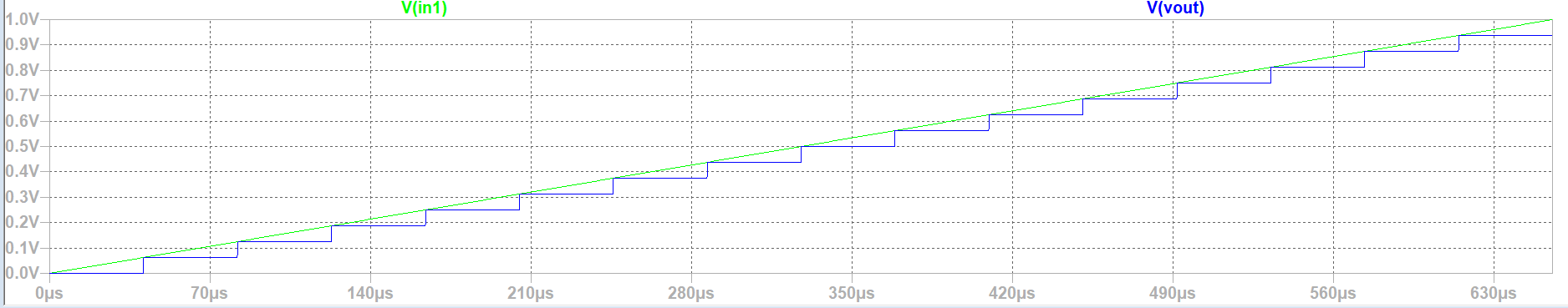# INL,DNL & FFT ANALYSIS OF ADC DAC

## LTSPICE INL, DNL FFT Analysis

• In 1st fig. we disable sine source and enable ramp then we use java script to do filtering
• Since we have ideal DAC ADC so we get ideal curve
• In 3rd fig. we have the extracted value
• In histogram test we need more time steps so we take 5.12E-6 steps.
• In 5th fig. we have odd number of cycles and inl and dnl of sine

•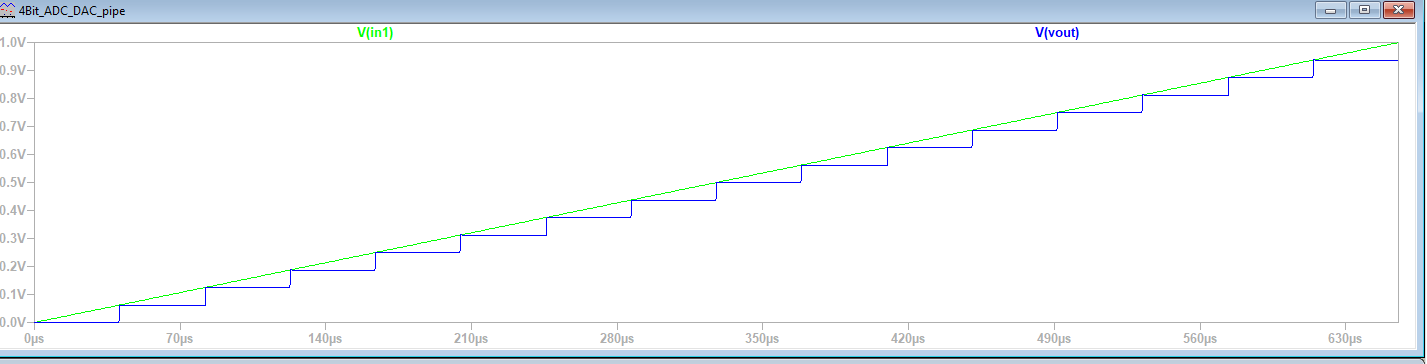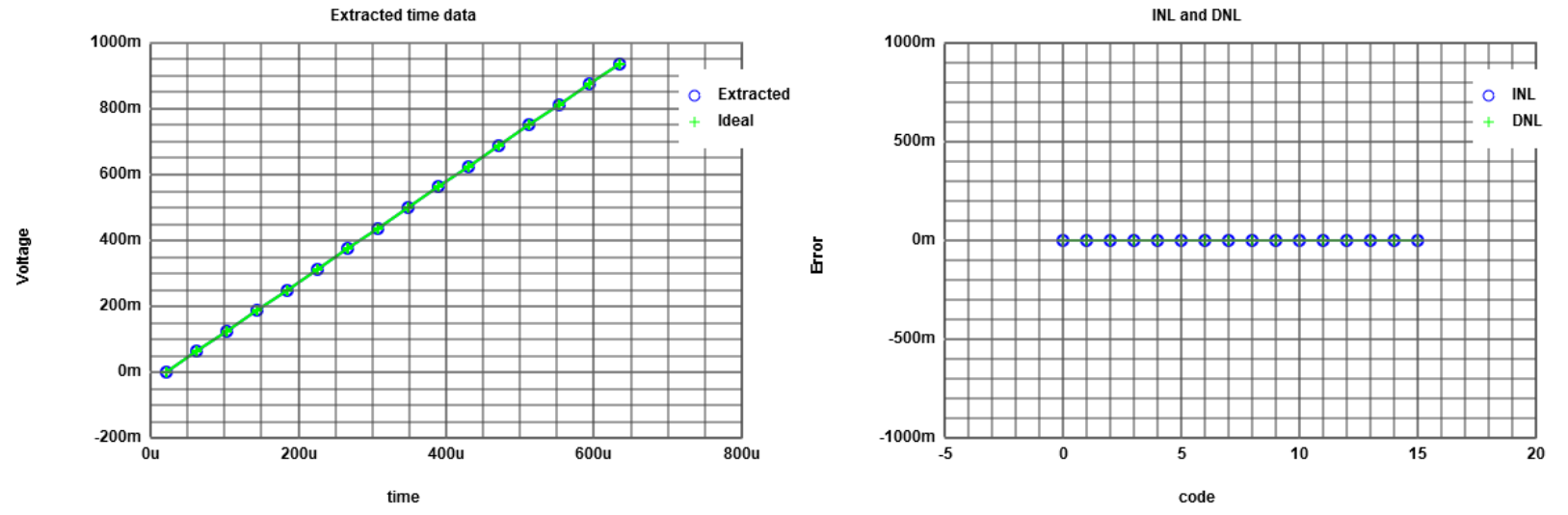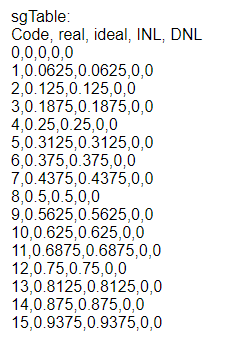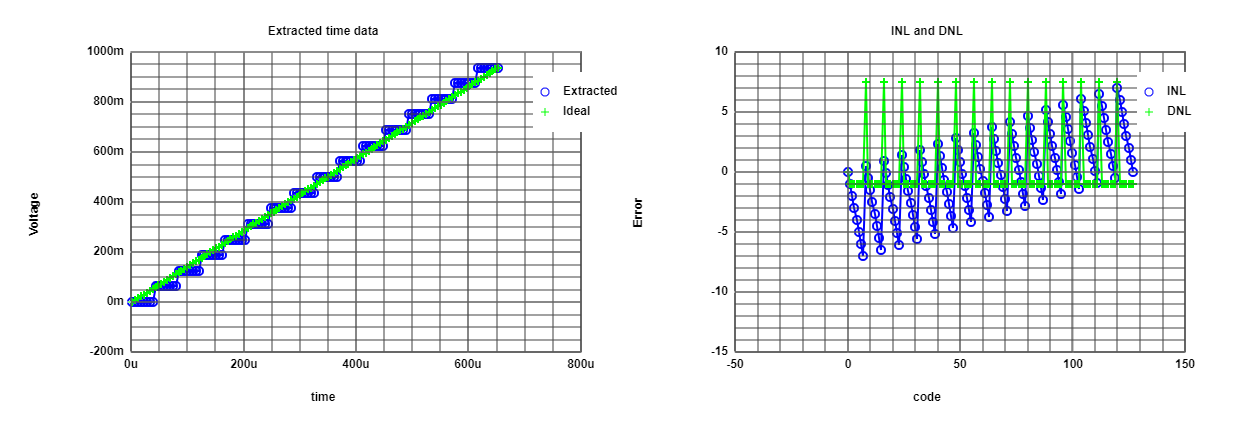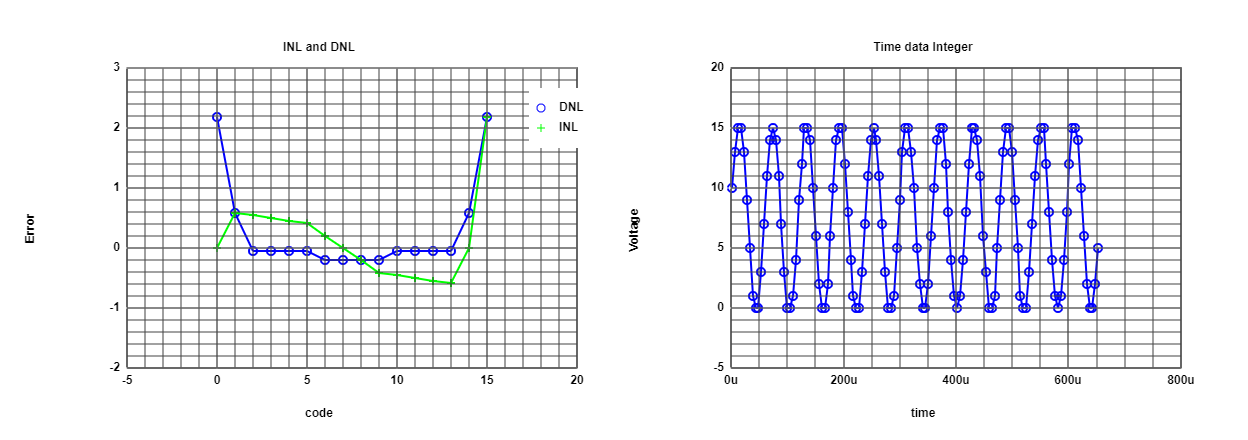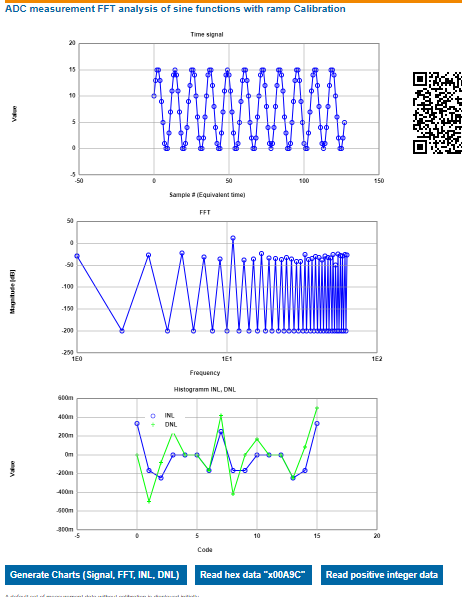# Simulation of ADC DAC with 4-bit R2R circuit

## LTSPICE INL, DNL FFT Analysis

• In fig.1 we analyze real R2R circuit using sine wave.
• In 2nd fig. we did fft analysis of sine function with ramp calibration.
• In 4th fig. we see error in ramp test.
• In 5th fig.we analyze signal to noise ratio.
• In 6th fig.we see jump in INL and DNL.
•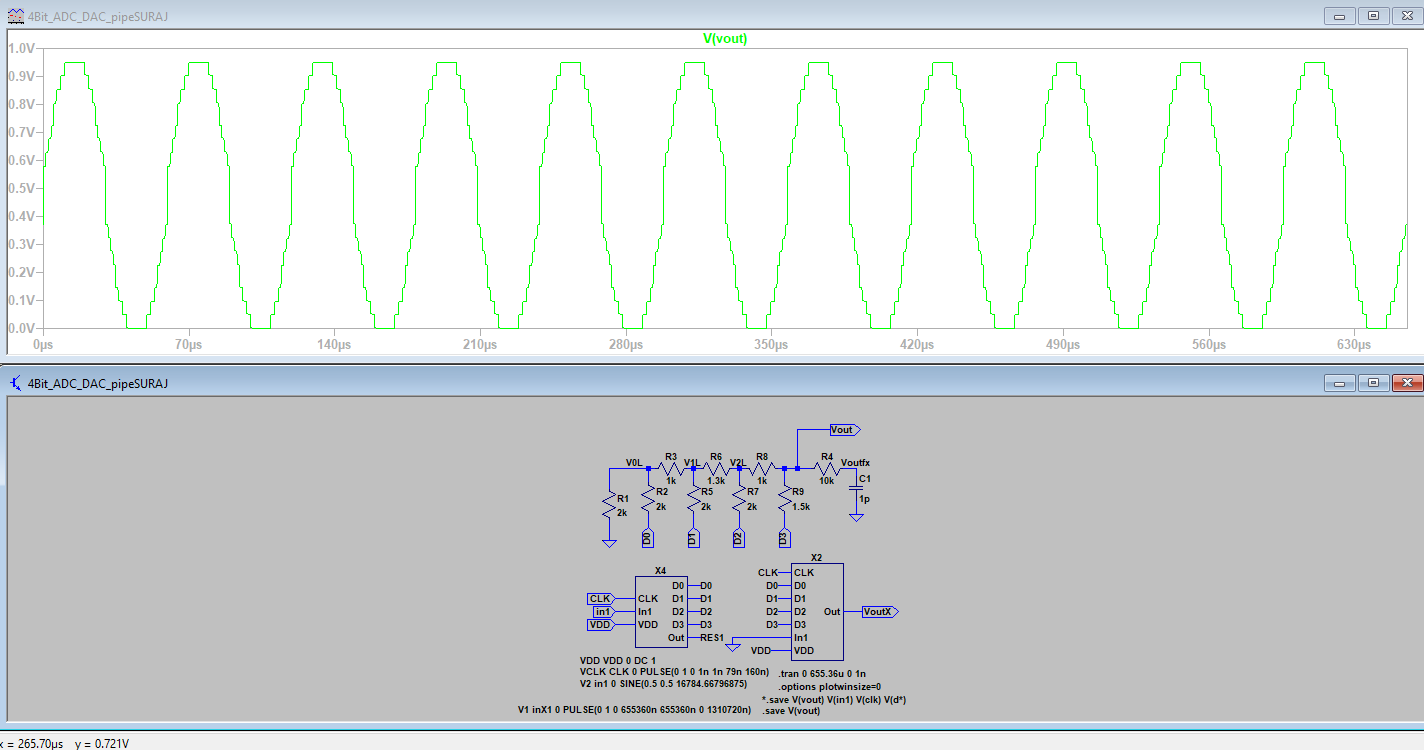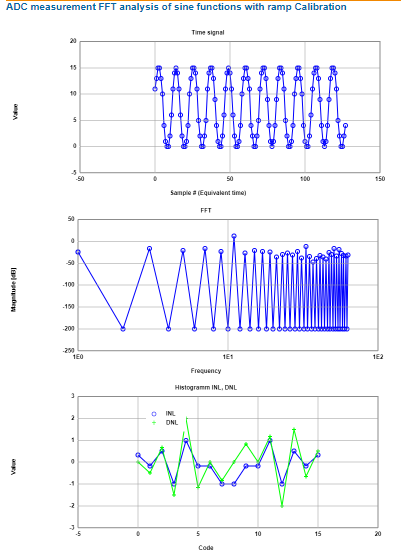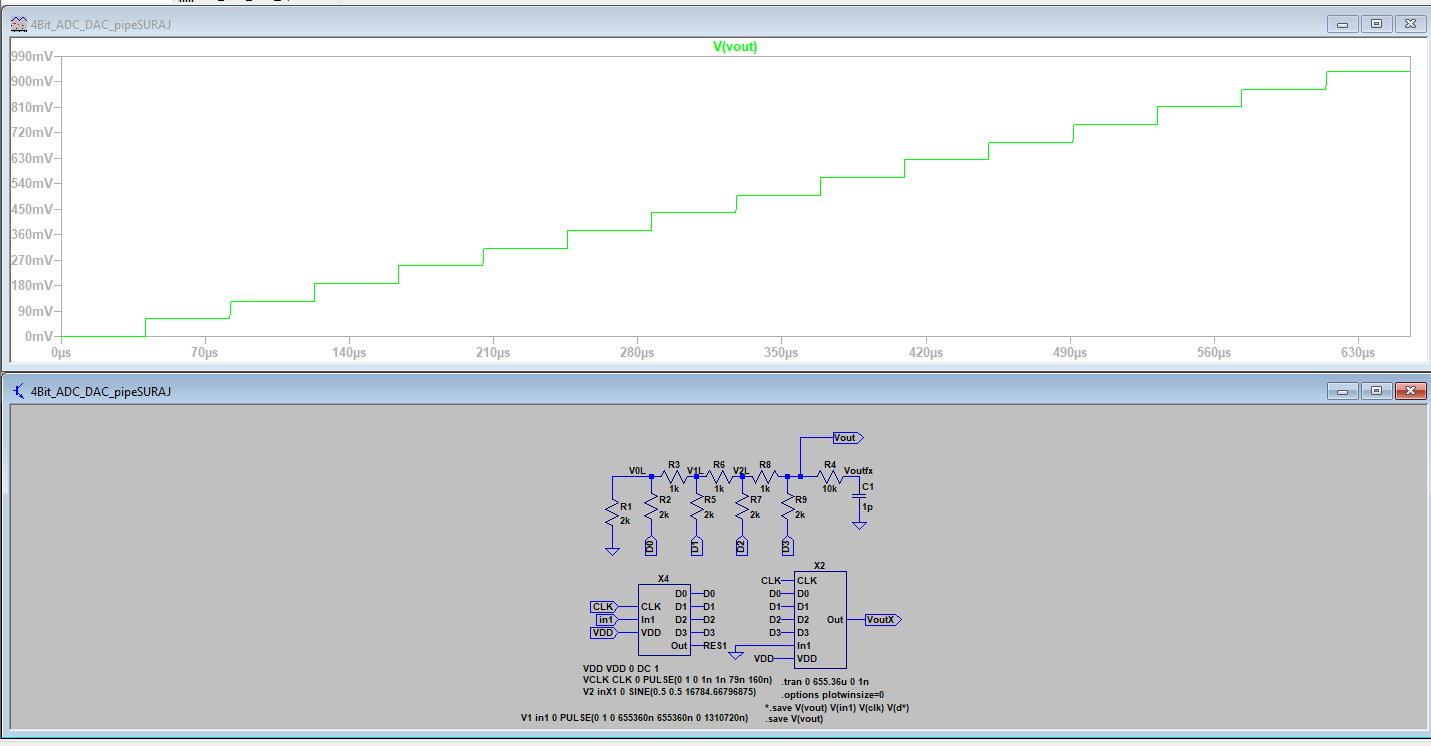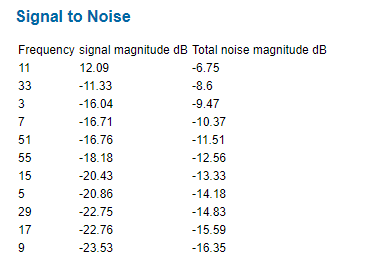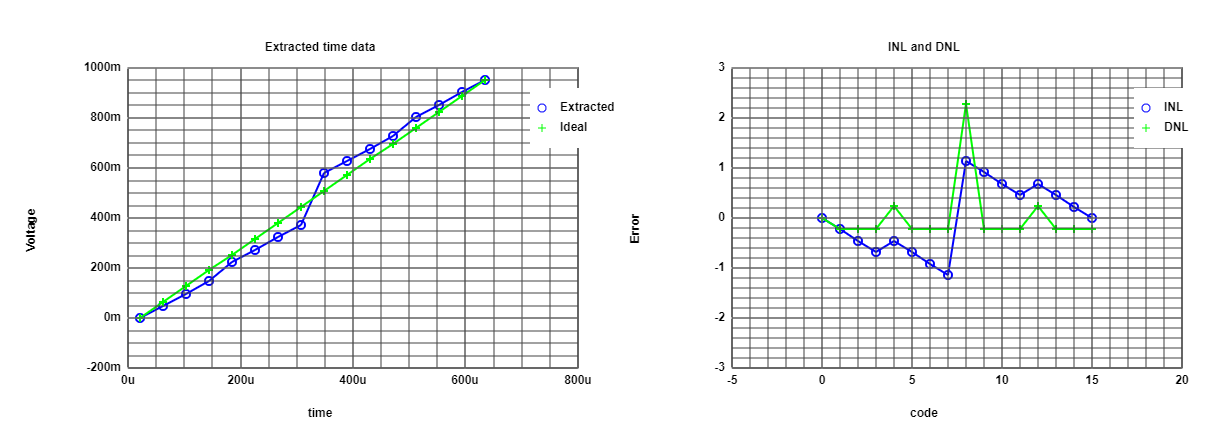# ADC DAC test simulation in LTSPICE with ramp

• .meas trans OUT00 FIND V(vout)AT=81.92us
• After simulation view spice error log will show the result
• After simulation "FILE" , "EXECUTE .MEAS script" generates the result
•